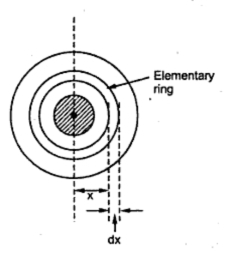### Insulation Resistance of a Cable

The Fig 1 shows the section of a single core cable which is insulated with the help of layer of an insulating material.Fig. 1  Single core cable

In such cables, the leakage current flows radially from centre towards the surface as shown in the Fig.1. Hence the cross-section of the path of such current is not constant but changes with its length. The resistance offered by cable to path of the leakage current is called an insulation resistance consider an elementary section of the cylindrical cable of radius x and the thickness dx as shown in the Fig. 2. Let us find the resistance of this elementary ring.
Let d = Diameter of core
r = d/2 = Radius of core
D = Diameter with sheath
R = D/2 = Radius of cable with sheathFig. 2   Elementary ring

As the leakage current flows radially outwards, the length along which the current flows in an elementary ring is dx. While the cross-sectional area perpendicular to the flow of current depends on the length of l of the cable.
Cross-section area = Surface area for length l of cable
= (2 π x) x l
Hence the resistance of this elementary cylindrical shell is,

where ρ = Resistivity of the insulating material
The total insulation resistance of the cable can be obtained by integrating the resistance of an elementary ring from inner radius upto the outer radius i.e. r to R.

This can be expressed interms of diameters as,

The value of Ri is always very high. The expression shows that the insulation resistance is inversely proportional to its length. So as the cable length increases, the insulation resistance decreases.
This shows that if two cables are joined in series then total length increases and hence their conductor resistances are in series giving higher resistance but insulation resistance are in parallel decreasing the effective insulation resistance. Thus if two cables are connected in parallel, conductor resistances get connected in parallel while the insulation resistance get connected in series.
1.